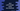# Java program to print triangle or reverse triangle using any character## Java program to print Triangle pattern using ‘star’ or any character :

In this tutorial, we will show you how to print a Triangle in Java. We can print the Triangle using any character like *,&,\$ etc.

### Sample program to print a Triangle in Java :

``````import java.util.Scanner;

public class Main {

public static void main(String[] args) {
Scanner sc = new Scanner(System.in);
int size = 0;
Character c;

System.out.println("Enter height of the triangle : ");
size = sc.nextInt();

System.out.println("Which character you want to use : ");
c = sc.next().charAt(0);

int i, j, k;

for (i = 0; i < size + 1; i++) {
for (j = size; j > i; j--) {
System.out.print(" ");
}
for (k = 0; k < (2 * i - 1); k++) {
System.out.print(c);
}
System.out.println();
}

}
}``````

### Output :

You need to enter the height of a triangle and the character you want to use for the triangle. It will then print the triangle like below :

``````Enter height of the triangle :
5
Which character you want to use :
*

*
***
*****
*******
*********``````

To understand this program, let me change the space with ’^’ . Now you can easily understand it by going through the for loops. If we replace the spaces with ’^’, it will look like :

``````^^^^^
^^^^*
^^^***
^^*****
^*******
*********``````

### Sample program to print reverse triangle in java :

Printing a reverse triangle looks same as above. Source :

``````import java.util.Scanner;

public class Main {

public static void main(String[] args) {
Scanner sc = new Scanner(System.in);
int size;
Character c;

System.out.println("Enter height of the triangle : ");
size = sc.nextInt();

System.out.println("Which character you want to use : ");
c = sc.next().charAt(0);

int i, j, k;

for (i = size; i > 0; i--) {
for (j = size; j > i; j--) {
System.out.print(" ");
}
for (k = 0; k < (i * 2 - 1); k++) {
System.out.print(c);
}
System.out.println();
}
}
}``````

### Sample Example :

``````Enter height of the triangle :
7
Which character you want to use :
\$
\$\$\$\$\$\$\$\$\$\$\$\$\$
\$\$\$\$\$\$\$\$\$\$\$
\$\$\$\$\$\$\$\$\$
\$\$\$\$\$\$\$
\$\$\$\$\$
\$\$\$
\$``````